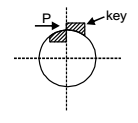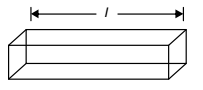## Machine Design Miscellaneous

#### Machine Design

1. Square key of side d/4 each and length l is used to transmit torque T from the shaft of diameter d to the hub of a pulley. Assuming the length of the key to be equal to the thickness of the pulley, the average shear stress developed in the key is given by

1. Force of the shaft circumference is given by

 P = T d/2

 ⇒ P = 2T dShearing area = width × length
 = d × l = ld 4 4Average shear stress = P Shearing area##### Correct Option: C

Force of the shaft circumference is given by

 P = T d/2

 ⇒ P = 2T dShearing area = width × length
 = d × l = ld 4 4Average shear stress = P Shearing area1. A solid circular shaft needs to be designed to transmit a torque of 50 N.m. If the allowable shear stress of the material is 140 MPa, assuming a factor of safety of 2, minimum allowable design diameter in mm is

1. Here, T = 50N – m
τallowable = 140 MPa
FOS = 2;

 τsafe = τallowable = 70 MPa FOS

 We know, T τsafe Zp

 Zp = πd3 16

 ⇒ d3 = 16 T π τsafe= 15.38 mm ≈ 16 mm

##### Correct Option: B

Here, T = 50N – m
τallowable = 140 MPa
FOS = 2;

 τsafe = τallowable = 70 MPa FOS

 We know, T τsafe Zp

 Zp = πd3 16

 ⇒ d3 = 16 T π τsafe= 15.38 mm ≈ 16 mm

1. A self-aligning ball bearing has a basic dynamic load rating (C10, for 106 revolutions) of 35 kN. If the equivalent radial load on the bearing is 45 kN, the expected life (in 106 revolutions) is

1.  Life (in Million Revolution) =Cq Pe

q → 3 = ball bearing
 q → 10 ⇒ Roller bearing 3

 Life =353 = 0.4705 Million Revolution 45

C → Dynamic load capacity = 35 KN
Pe equivalent load = 45 KN

##### Correct Option: A

 Life (in Million Revolution) =Cq Pe

q → 3 = ball bearing
 q → 10 ⇒ Roller bearing 3

 Life =353 = 0.4705 Million Revolution 45

C → Dynamic load capacity = 35 KN
Pe equivalent load = 45 KN

1. A butt weld Joint is developed on steel plates having yield and ultimate tensile strength of 500 MPa and 700 MPa, respectively. The thickness of the plates is 8 mm and width is 20 mm. Improper selection of welding parameters caused an undercut of 3 mm depth along the weld. The maximum transverse tensile load (in kN) carrying capacity of the developed weld Joint is _________.

1. Pt = (st) (tunder cut) L
= 700 × 20 × (8 – 3)
= 700 × 100
= 70 kN

##### Correct Option: A

Pt = (st) (tunder cut) L
= 700 × 20 × (8 – 3)
= 700 × 100
= 70 kN

1. A fillet welded Joint is subjected to transverse loading F as shown in figure. Both legs of the fillets are of 10 mm size and the weld length is 30 mm. If the allowable shear stress of the weld is 94 MPa, considering the minimum throat of the weld, the maximum allowable transverse load in kN is1. P = 0.707 sl. τallowable
= 0.707 × 10 × 30 × 94
= 19937.4N
= 19.934 kN

##### Correct Option: C

P = 0.707 sl. τallowable
= 0.707 × 10 × 30 × 94
= 19937.4N
= 19.934 kN